# When do we use vector multiplication

Products from vectors

There are three different ways of multiplying vectors:

• First, multiplying a vector by multiplying it by a real number,
• second, the scalar multiplication, in which the result is a real number (i.e. a scalar),
• and thirdly, vector multiplication, the result of which is a vector.
So let's go in sequence and first examine the multiplication of a vector.

Multiplying a vector

If a vector is multiplied by a real number, then all three coordinates of the vector must be multiplied by that number.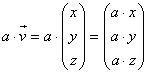Using numbers, it looks like this: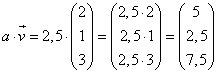Danger!!! If as a factor negative numbers are used, the direction of the vector is reversed; a multiplication by
-1 generates the Opposite vector for a given vector (see subtraction of vectors)!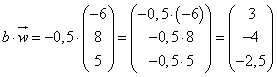The dot product of two vectors

The second way to multiply vectors is with the scalar product. As the name suggests, the result in this case is a scalar and not a vector. Nevertheless, the scalar product is justified, because the result has a peculiarity that makes the scalar product interesting. In general, we calculate the scalar product by multiplying the coordinates of the vectors in pairs and adding the products:Note that this multiplication is not by a point but by a small circle is symbolized! Let us now look at two examples with concrete numerical values: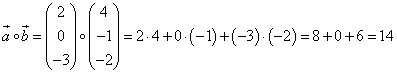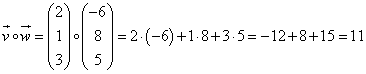But what is special about the results? As a third example, let's look at two vectors that are perpendicular to each other: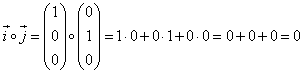We immediately see that the scalar product is zero - just like that cosine of the angle of 90 ° !!! More detailed investigations then also show the following connection:For the above example we get: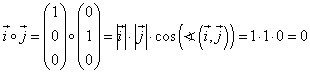This knowledge now allows us to determine the angle that two vectors enclose! To do this, we rearrange the above general relationship to the cosine of the angle:The animation explains the multiplication of vectors and the scalar multiplication of vectors. Start a - Shows the multiplication of the vector v (dark blue) by the number 2.5. Start b - Shows the multiplication of the vector w (light blue) by the number -0.5. Note that the direction of the vector changes here! Start c - Shows how the angle alpha (red) between the vectors v and w can be determined with the help of the scalar product.

[Exercises] [back to overview] [cross product]